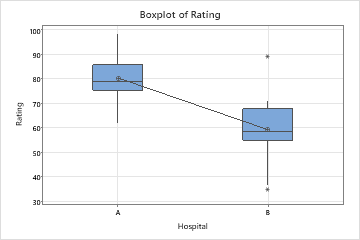# Interpret the key results for 2-Sample t

Complete the following steps to interpret a 2-sample t-test. Key output includes the estimate for difference, the confidence interval, the p-value, and several graphs.

## Step 1: Determine a confidence interval for the difference in population means

First, consider the difference in the sample means and then examine the confidence interval.

The difference is an estimate of the difference in the population means. Because the difference is based on sample data and not on the entire population, it is unlikely that the sample difference equals the population difference. To better estimate the population difference, use the confidence interval for the difference.

The confidence interval provides a range of likely values for the difference between two population means. For example, a 95% confidence level indicates that if you take 100 random samples from the population, you could expect approximately 95 of the samples to produce intervals that contain the population difference. The confidence interval helps you assess the practical significance of your results. Use your specialized knowledge to determine whether the confidence interval includes values that have practical significance for your situation. If the interval is too wide to be useful, consider increasing your sample size. For more information, go to Ways to get a more precise confidence interval.

## Step 2: Determine whether the difference is statistically significant

To determine whether the difference between the population means is statistically significant, compare the p-value to the significance level. Usually, a significance level (denoted as α or alpha) of 0.05 works well. A significance level of 0.05 indicates a 5% risk of concluding that a difference exists when there is no actual difference.
P-value ≤ α: The difference between the means is statistically significantly (Reject H0)
If the p-value is less than or equal to the significance level, the decision is to reject the null hypothesis. You can conclude that the difference between the population means does not equal the hypothesized difference. If you did not specify a hypothesized difference, Minitab tests whether there is no difference between the means (Hypothesized difference = 0). Use your specialized knowledge to determine whether the difference is practically significant. For more information, go to Statistical and practical significance.
P-value > α: The difference between the means is not statistically significant (Fail to reject H0)
If the p-value is greater than the significance level, the decision is to fail to reject the null hypothesis. You do not have enough evidence to conclude that the difference between the population means is statistically significant. You should make sure that your test has enough power to detect a difference that is practically significant. For more information, go to Power and Sample Size for 2-Sample t.

## Step 3: Check your data for problems

Problems with your data, such as skewness and outliers can adversely affect your results. Use the graphs to look for skewness (by examining the spread of each sample) and to identify potential outliers.

When data are skewed, the majority of the data are located on the high or low side of the graph. Often, skewness is easiest to detect with a histogram or boxplot.The boxplot with right-skewed data shows wait times. Most of the wait times are relatively short, and only a few wait times are long. The boxplot with left-skewed data shows failure time data. A few items fail immediately, and many more items fail later.

Data that are severely skewed can affect the validity of the p-value if your samples are small (either sample is less than 15 values). If your data are severely skewed and you have a small sample, consider increasing your sample size.Sample outputIn these boxplots, the data for Hospital B appear to be severely skewed.

### Identify outliers

Outliers, which are data values that are far away from other data values, can strongly affect the results of your analysis. Often, outliers are easiest to identify on a boxplot.